Updating search results...

# 28 Results

View
Selected filters:
• Trigonometry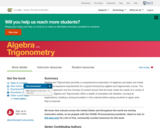Unrestricted Use
CC BY
Rating
0.0 stars

Algebra and Trigonometry provides a comprehensive exploration of algebraic principles and meets scope and sequence requirements for a typical introductory algebra and trigonometry course. The modular approach and the richness of content ensures that the book meets the needs of a variety of courses. Algebra and Trigonometry offers a wealth of examples with detailed, conceptual explanations, building a strong foundation in the material before asking students to apply what they’ve learned.

Subject:
Algebra
Mathematics
Trigonometry
Material Type:
Textbook
Provider:
Rice University
Provider Set:
OpenStax College
Author:
David Lippman
Jay Abramson
Jean-Marie Magnier
Melonie Rasmussen
Nicholas Belloit
Rachael Gross
Rick Norwood
Valeree Falduto
01/29/2015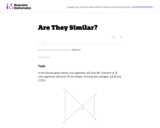Unrestricted Use
CC BY
Rating
0.0 stars

In this problem, students are given a picture of two triangles that appear to be similar, but whose similarity cannot be proven without further information. Asking students to provide a sequence of similarity transformations that maps one triangle to the other focuses them on the work of standard G-SRT.2, using the definition of similarity in terms of similarity transformations.

Subject:
Geometry
Mathematics
Trigonometry
Material Type:
Activity/Lab
Provider:
Illustrative Mathematics
Provider Set:
Illustrative Mathematics
Author:
Illustrative Mathematics
05/01/2012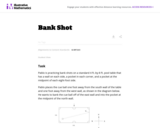Unrestricted Use
CC BY
Rating
0.0 stars

This task asks students to use similarity to solve a problem in a context that will be familiar to many, though most students are accustomed to using intuition rather than geometric reasoning to set up the shot.

Subject:
Geometry
Mathematics
Trigonometry
Material Type:
Activity/Lab
Provider:
Illustrative Mathematics
Provider Set:
Illustrative Mathematics
Author:
Illustrative Mathematics
08/21/2012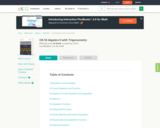Unrestricted Use
CC BY
Rating
0.0 stars

This textbook covers Algebra II and Trigonometry topics with chapters on equations and inequalities, linear equations and functions, systems of linear equations and inequalities, matrices, quadratic functions and more.

Subject:
Algebra
Mathematics
Trigonometry
Material Type:
Textbook
Provider:
CK-12 Foundation
Provider Set:
CK-12 FlexBook
07/13/2021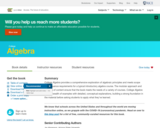Unrestricted Use
CC BY
Rating
0.0 stars

College Algebra provides a comprehensive exploration of algebraic principles and meets scope and sequence requirements for a typical introductory algebra course. The modular approach and richness of content ensure that the book meets the needs of a variety of courses. College Algebra offers a wealth of examples with detailed, conceptual explanations, building a strong foundation in the material before asking students to apply what theyve learned.

Subject:
Algebra
Mathematics
Trigonometry
Material Type:
Textbook
Provider:
Rice University
Provider Set:
OpenStax College
Author:
David Lippman
Jay Abramson
Jean-Marie Magnier
Melonie Rasmussen
Nicholas Belloit
Rachael Gross
Rick Norwood
Valeree Falduto
01/29/2015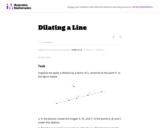Unrestricted Use
CC BY
Rating
0.0 stars

This task gives students the opportunity to verify that a dilation takes a line that does not pass through the center to a line parallel to the original line, and to verify that a dilation of a line segment (whether it passes through the center or not) is longer or shorter by the scale factor.

Subject:
Geometry
Mathematics
Trigonometry
Material Type:
Activity/Lab
Provider:
Illustrative Mathematics
Provider Set:
Illustrative Mathematics
Author:
Illustrative Mathematics
05/01/2012Conditional Remix & Share Permitted
CC BY-NC-SA
Rating
0.0 stars

This tasks examines how to calculate the area of an equilateral triangle using high school geometry.

Subject:
Geometry
Mathematics
Trigonometry
Material Type:
Activity/Lab
Provider:
Illustrative Mathematics
Provider Set:
Illustrative Mathematics
Author:
Illustrative Mathematics
01/20/2013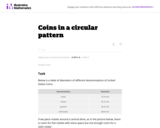Unrestricted Use
CC BY
Rating
0.0 stars

This task complements ``Seven Circles'' I, II, and III. This is a hands-on activity which students could work on at many different levels and the activity leads to many interesting questions for further investigation.

Subject:
Geometry
Mathematics
Trigonometry
Material Type:
Activity/Lab
Provider:
Illustrative Mathematics
Provider Set:
Illustrative Mathematics
Author:
Illustrative Mathematics
08/21/2012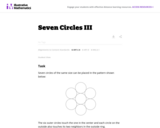Unrestricted Use
CC BY
Rating
0.0 stars

This task provides an opportunity to model a concrete situation with mathematics. Once a representative picture of the situation described in the problem is drawn (the teacher may provide guidance here as necessary), the solution of the task requires an understanding of the definition of the sine function. When the task is complete, new insight is shed on the ``Seven Circles I'' problem which initiated this investigation as is noted at the end of the solution.

Subject:
Geometry
Mathematics
Trigonometry
Material Type:
Activity/Lab
Provider:
Illustrative Mathematics
Provider Set:
Illustrative Mathematics
Author:
Illustrative Mathematics
08/21/2012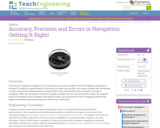Educational Use
Rating
0.0 stars

In this lesson, students will investigate error. As shown in earlier activities from navigation lessons 1 through 3, without an understanding of how errors can affect your position, you cannot navigate well. Introducing accuracy and precision will develop these concepts further. Also, students will learn how computers can help in navigation. Often, the calculations needed to navigate accurately are time consuming and complex. By using the power of computers to do calculations and repetitive tasks, one can quickly see how changing parameters likes angles and distances and introducing errors will affect their overall result.

Subject:
Applied Science
Engineering
Geometry
Mathematics
Trigonometry
Material Type:
Activity/Lab
Lesson Plan
Provider:
TeachEngineering
Provider Set:
TeachEngineering
Author:
Janet Yowell
Jeff White
Malinda Schaefer Zarske
Matt Lippis
09/18/2014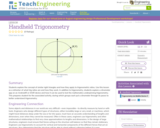Educational Use
Rating
0.0 stars

Students explore the concept of similar right triangles and how they apply to trigonometric ratios. Use this lesson as a refresher of what trig ratios are and how they work. In addition to trigonometry, students explore a clinometer app on an Android® or iOS® device and how it can be used to test the mathematics underpinning trigonometry. This prepares student for the associated activity, during which groups each put a clinometer through its paces to better understand trigonometry.

Subject:
Applied Science
Engineering
Mathematics
Trigonometry
Material Type:
Lesson Plan
Provider:
TeachEngineering
Provider Set:
TeachEngineering
Author:
Scott Burns
09/18/2014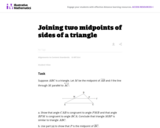Unrestricted Use
CC BY
Rating
0.0 stars

This task is closely related to very important material about similarity and ratios in geometry.

Subject:
Geometry
Mathematics
Trigonometry
Material Type:
Activity/Lab
Provider:
Illustrative Mathematics
Provider Set:
Illustrative Mathematics
Author:
Illustrative Mathematics
01/21/2013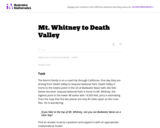Unrestricted Use
CC BY
Rating
0.0 stars

The purpose of this task is to engage students in an open-ended modeling task that uses similarity of right triangles, and also requires the use of technology.

Subject:
Geometry
Mathematics
Trigonometry
Material Type:
Activity/Lab
Provider:
Illustrative Mathematics
Provider Set:
Illustrative Mathematics
Author:
Illustrative Mathematics
02/04/2013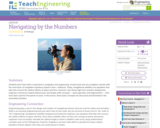Educational Use
Rating
0.0 stars

In this lesson, students will learn that math is important in navigation and engineering. Ancient land and sea navigators started with the most basic of navigation equations (Speed x Time = Distance). Today, navigational satellites use equations that take into account the relative effects of space and time. However, even these high-tech wonders cannot be built without pure and simple math concepts basic geometry and trigonometry that have been used for thousands of years. In this lesson, these basic concepts are discussed and illustrated in the associated activities.

Subject:
Applied Science
Engineering
Geometry
Mathematics
Trigonometry
Material Type:
Activity/Lab
Lesson Plan
Provider:
TeachEngineering
Provider Set:
TeachEngineering
Author:
Janet Yowell
Jeff White
Malinda Schaefer Zarske
09/18/2014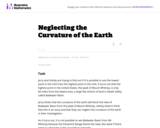Unrestricted Use
CC BY
Rating
0.0 stars

This task applies geometric concepts, namely properties of tangents to circles and of right triangles, in a modeling situation. The key geometric point in this task is to recognize that the line of sight from the mountain top towards the horizon is tangent to the earth. We can then use a right triangle where one leg is tangent to a circle and the other leg is the radius of the circle to investigate this situation.

Subject:
Geometry
Mathematics
Trigonometry
Material Type:
Activity/Lab
Provider:
Illustrative Mathematics
Provider Set:
Illustrative Mathematics
Author:
Illustrative Mathematics
03/04/2013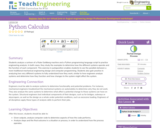Educational Use
Rating
0.0 stars

Students analyze a cartoon of a Rube Goldberg machine and a Python programming language script to practice engineering analysis. In both cases, they study the examples to determine how the different systems operate and the function of each component. This exercise in juxtaposition enables students to see the parallels between a more traditional mechanical engineering design and computer programming. Students also gain practice in analyzing two very different systems to fully understand how they work, similar to how engineers analyze systems and determine how they function and how changes to the system might affect the system.

Subject:
Applied Science
Education
Engineering
Mathematics
Trigonometry
Material Type:
Lesson Plan
Provider:
TeachEngineering
Provider Set:
TeachEngineering
Author:
Brian Sandall
Scott Burns
09/18/2014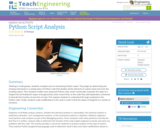Educational Use
Rating
0.0 stars

Working in small groups, students complete and run functioning Python codes. They begin by determining the missing commands in a sample piece of Python code that doubles all the elements of a given input and sums the resulting values. Then students modify more advanced Python code, which numerically computes the slope of a tangent line by finding the slopes of progressively closer secant lines; to this code they add explanatory comments to describe the function of each line of code. This requires students to understand the logic employed in the Python code. Finally, students make modifications to the code in order to find the slopes of tangents to a variety of functions.

Subject:
Applied Science
Education
Engineering
Mathematics
Trigonometry
Material Type:
Activity/Lab
Provider:
TeachEngineering
Provider Set:
TeachEngineering
Author:
Brian Sandall
Scott Burns
09/18/2014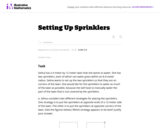Unrestricted Use
CC BY
Rating
0.0 stars

This modeling task involves several different types of geometric knowledge and problem-solving: finding areas of sectors of circles (G-C.5), using trigonometric ratios to solve right triangles (G-SRT.8), and decomposing a complicated figure involving multiple circular arcs into parts whose areas can be found (MP.7).

Subject:
Geometry
Mathematics
Trigonometry
Material Type:
Activity/Lab
Provider:
Illustrative Mathematics
Provider Set:
Illustrative Mathematics
Author:
Illustrative Mathematics
05/01/2012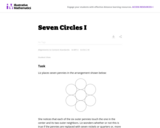Unrestricted Use
CC BY
Rating
0.0 stars

This task is intended to help model a concrete situation with geometry. Placing the seven pennies in a circular pattern is a concrete and fun experiment which leads to a genuine mathematical question: does the physical model with pennies give insight into what happens with seven circles in the plane?

Subject:
Geometry
Mathematics
Trigonometry
Material Type:
Activity/Lab
Provider:
Illustrative Mathematics
Provider Set:
Illustrative Mathematics
Author:
Illustrative Mathematics
08/20/2012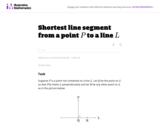Unrestricted Use
CC BY
Rating
0.0 stars

This is a foundational geometry task designed to provide a route for students to develop some fundamental geometric properties that may seem rather obvious at first glance. In this case, the fundamental property in question is that the shortest path from a point to a line meets the line at a right angle, which is crucial for many further developments in the subject.

Subject:
Geometry
Mathematics
Trigonometry
Material Type:
Activity/Lab
Provider:
Illustrative Mathematics
Provider Set:
Illustrative Mathematics
Author:
Illustrative Mathematics